The Destructuring Assignment syntax is a way to assign the values from Arrays and Structs(Complex Objects), into distinct variables.

Syntax:

Array Destructuring
`[variable1, variable2, ............, variableN] = [value1, value2, ......., valueN];`

Object Destructuring
`({key1, key2, ............, keyN} = {key1:value1, key2:value2, ......., keyN:valueN});`

DeStructuring is a very powerful feature of a language. Consider the scenarios where a developer is interacting with data. The data is coming from an API in JSON format and he only wants to extract certain values and ignore others.

Conventional way of doing it is to store the object and then retrieve it using the JSON Object API calls. However using DeStructuring, it becomes very simple, where just by writing a line of code, one can extract the relevant attributes and choose to ignore the other ones.

Note: The above defined syntax of [variable1, variable2, …………, variableN] [value1, value2, ……., valueN] is written for the sake of simplicity. This syntax can be as complex as possible.

For example here the LVAL i.e [variable1, variable2, …………, variableN], can be substituted with complex  values like Array/Struct elements or even a complex Object.

Similarly the RVAL i.e [value1, value2, ……., valueN], can be substituted by Array/Struct elements as well as Array/Struct References or even a complex Object.

Lets get into more details and try to simulate the different scenarios through examples and see how we can write some short, concise and more powerful syntax using this feature.

• Multi-Initializer Statements with same #LVAL and #RVAL
`<``cfscript``>`
`[a, b] = [10, 20];`
`writeDump(a);`
`writeDump(b);`
`</``cfscript``>`

• Multi-Initializer Statements with RVAL as an Array Reference
`<``cfscript``>`
`arr = [100, 200, 300];`
`[a, b, c] = arr;`
`writeDump(a);`
`writeDump(b);`
`writeDump(c);`
`</``cfscript``>`

• Multi-Initializer Statements by ignoring extra RVALs
`<``cfscript``>`
`arr = [100, 200, 300];`
`[firstVal, secondVal] = arr;`

`writeDump(firstVal);`
`writeDump(secondVal);`
`</``cfscript`

• Multi-Initializer Statements to ignore some values from RVAL
`<``cfscript``>`
`function f() {`
`return [1, 2, 3];`
`}`
`[firstValue, , lastValue] = f();`

`writeDump(firstValue);`
`writeDump(lastValue);`
`</``cfscript``>`

• Multi-Initializer Statements using Rest Operator(…)
`<``cfscript``>`
`arr = [100, 200, 300, 400, 500];`
`[firstVal, secondVal, ...rest] = arr;`

`writeDump(firstVal);`
`writeDump(secondVal);`
`writeDump(rest);    //assigns [300, 400, 500] to rest`
`</``cfscript``>`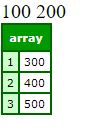• Complex Multi-Initializer Statements
`<``cfscript``>`
`[foo, [[bar], baz]] = [61,[, 23]];`
`writeDump(foo);`
`writeDump(bar);`
`writeDump(baz);`
`</``cfscript``>`
• Swapping Values
`<``cfscript``>`
`   ``a=1;`
`   ``b=2;`
`   ``[a,b]=[b,a];`
`   ``writeoutput``(a)`
`   ``writeoutput``(b)`
`   ``writeOutput``(``"<br>"``);`
`</``cfscript``>`

`<``cfscript``>`
`   ``arr = [1,2,3];`
`   ``[arr, arr] = [arr, arr];`
`   ``writedump(arr);`
`</``cfscript``>`

Object Destructuring : Up till now, we have mostly seen the examples of ArrayInitializers as well as complex DeStructuring. DeStructuring is also supported for Structs as well as Complex Objects. Lets take some examples to simulate Object Destructuring and how we can leverage this functionality for Structs.

• Multi-Initializer Statements with same #LVAL and #RVAL
`<``cfscript``>`
`({ a, b, c } = { a: 10, b: {CF_PREV:``"2018"``,CF_CURR:``"2020"``}, c: [21,22,34] })`
`writeDump(a);`
`writeDump(b);`
`writeDump(c);`
`</``cfscript``>`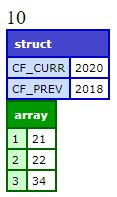• Multi-Initializer Statements using Object Reference
`<``cfscript``>`
`student = {`
`firstname: ``'Glad'``,`
`lastname: ``'Chinda'``,`
`country: ``'Nigeria'`
`};`

`// Reassign firstname ``and` `lastname using destructuring`
`({ firstname, lastname } = student);`

`writeDump(firstname);`
`writeDump(lastname);`
`</``cfscript``>`
• Multi-Initializer Statements with LVAL Object having default Values
`<``cfscript``>`
`student = {`
`    ``firstname: ``'Glad'``,`
`    ``lastname: ``'Chinda'``,`
`    ``country: ``'Nigeria'`
`};`

`// Reassign firstname ``and` `lastname using destructuring`
`({ firstname, lastname, age =25 } = student);`

`writeDump(firstname);`
`writeDump(lastname);`
`writeDump(age);`
`</``cfscript``>`
• Nested Object Destructuring: Using this one can initialize attributeValues from childObjects within ParentObject.
`<``cfscript``>`
`person = {`
`    ``name: ``'John Doe'``,`
`    ``age: 25,`
`    ``location: {`
`        ``country: ``'Canada'``,`
`        ``city: ``'Vancouver'``,`
`        ``coordinates: [49.2827, -123.1207]`
`    ``}`
`};`

`({name, location: {country, city, coordinates: [lat, lng]}} = person);`

`writeDump(name);`
`writeDump(city)`
`writeDump(lat);`
`writeDump(lng);`
`//writeDump(coordinates);`
`writeDump(country);`
`</``cfscript``>`

The Output of the above code-snippet is as shown below.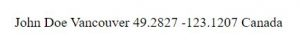• Rest Operator Support in Objects Destructuring Multi-Initializer Statements
`<``cfscript``>`
`({as, bs, ...rest} = {as: 40, bs: [111,122,133], c:23, d:{CFPREV:``"2018"``,CF_CURR:``"2020"``}, e:[21,22,34]});`
`writeDump(as);`
`writeDump(bs);`
`writeDump(rest);`
`</``cfscript``>`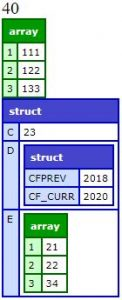• Ignoring RVAL in Objects Destructuring
`<``cfscript``>`
`({ a, , c } = { a: 10, b: {CFPREV:``"2018"``,CF_CURR:``"2020"``}, c: [21,22,34] })`
`writeDump(a);`
`writeDump(c);`
`</``cfscript``>`

DeStructuring is also supported for local/final and static variables. Lets take some examples to explore the functionality in local/final and static variables. Note () are not required for local/final variables declaration.
• Destructuring Support in local variables using var keyword
`<``cfscript``>`

`function func1(){`
`[x, y, z] = [23, 24, 25];`
`var [foo, [[bar], baz]] = [61, [, 23]];`
`var [a, , , p] = [``"A"``, ``"B"``, ``"C"``, ``"P"``];`
`writeDump(foo);`
`writeDump(bar);`
`writeDump(baz);`
`writeDump(y);`
`writeDump(p);`
`}`

`func1();`

`writeDump(x);`

`//writeDump(p); // Will throw an Exception`
`//writeDump(foo);   // Will throw an Exception`
`//writeDump(bar);   //Will throw an Exception`
`</``cfscript``>`

`<!---`
`     ``Code-snippet for Complex Objects`
`--->`

`<``cfscript``>`
`function func(){`
`person = {`
`    ``name: ``'John Doe'``,`
`    ``age: 25,`
`    ``location: {`
`        ``country: ``'Canada'``,`
`        ``city: ``'Vancouver'``,`
`        ``coordinates: [49.2827, -123.1207]`
`    ``}`
`};`

`var {as, bs, ...rest} = {as: 40, bs: [111,122,133], c:23, d:{CFPREV:``"2018"``,CF_CURR:``"2020"``}, e:[21,22,34]};`

`writeDump(rest);`
`writeDump(bs);`
`writeDump(as);`
`}`

`func();`

`//writeDump(rest); // Variable REST is undefined.`
`//writeDump(bs); // Variable BS is undefined.`

`writeDump(person);`
`</``cfscript``>`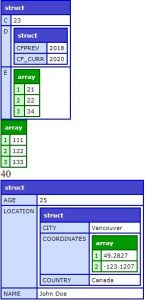• Destructuring Support using Final keyword in Global as well as Local Scope
`<``cfscript``>`
`final {as, bs, ...rest} = {as: 40, bs: [111,122,133], c:23, d:{CFPREV:``"2018"``,CF_CURR:``"2020"``}, e:[21,22,34]};`
`//as = 3; //should throw Final variable REST value change exception.`
`//rest = ``"123"``; //should throw Final variable REST value change exception.`

`writeDump(as);`
`writeDump(bs);`
`writeDump(rest);`

`</``cfscript``>`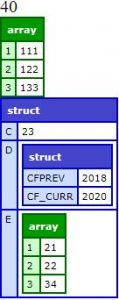`<!---`
`Code-snippet for final var support`
`--->`

`<``cfscript``>`
`function func(){`
`person = {`
`name: ``'John Doe'``,`
`age: 25,`
`location: {`
`country: ``'Canada'``,`
`city: ``'Vancouver'``,`
`coordinates: [49.2827, -123.1207]`
`}`
`};`

`var {as, bs, ...rest} = {as: 40, bs: [111,122,133], c:23, d:{CFPREV:``"2018"``,CF_CURR:``"2020"``}, e:[21,22,34]};`
`final var {as1, bs1, ...rest1} = {as1: 40, bs1: [111,122,133], c:23, d:{CFPREV:``"2018"``,CF_CURR:``"2020"``}, e:[21,22,34]}`

`writeDump(rest);`
`writeDump(bs);`
`writeDump(as1);`

`//as1 = [23,24,35]; // Final variable AS1 value change exception.`

`writeDump(as1);`
`}`

`func();`

`//writeDump(rest); // Variable REST is undefined.`
`//writeDump(bs); // Variable REST is undefined.`
`writeDump(person);`
`</``cfscript``>`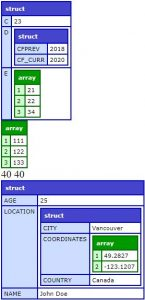`<!---`
`     ``Code-snippet for Complex Array Initializers`
`--->`

`<``cfscript``>`

`final [ax, ay, az] = [23, 24, 25];`
`//final var [ax, ay, az] = [23, 24, 25]; // The local variable ax cannot be declared outside of a function.`

`function func1(){`

`final [x, y, z] = [23, 24, 25];`

`final var [foo, [[bar], baz]] = [61, [, 23]];`
`final var [a, , , p] = [``"A"``, ``"B"``, ``"C"``, ``"P"``];`
`final var [b, c, ...rest] = [10, 20, 30, 40, 50];`

`//foo =234; // Should throw Final Variable foo Value Change Exception`
`//baz = 234; // Should throw Final Variable baz Value Change Exception`
`//p =3; // Should throw Final Variable p Value Change Exception`
`//rest = [4, 5, 6]; // Should throw Final Variable rest Value Change Exception`
`// x = 25; // Should throw Final Variable X Value Change Exception`

`writeDump(foo);`
`writeDump(bar);`
`writeDump(baz);`
`writeDump(y);`
`writeDump(p);`
`writeDump(rest);`

`}`

`func1();`

`//x=25; // Should throw Final Variable X Value Change Exception`
`//ax =34; // Should throw Final Variable AX Value Change Exception`

`writeDump(x);`

`//writeDump(p); // Will throw an Exception`
`//writeDump(foo); // Will throw an Exception`
`//writeDump(bar); //Will throw an Exception`
`//writeDump(rest); //Will throw an Exception`

`</``cfscript``>`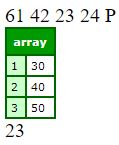• Object Destructuring static keyword support : Object Destructuring can also be leveraged in components using the static keyword. A sample code-snippet is as shown below.
 `=========================` `Sample.cfc` `=========================` `component {` `    ``static [a, , , , c] = [10, 22, 33, 44, 55];` `    ``static {as, bs, ...rest} = {as: 40, bs: [111,122,133], c:23, d:{CFPREV:``"2018"``,CF_CURR:``"2020"``}, e:[21,22,34]};` `}`     `=========================` `test.cfm` `=========================`   `<``cfscript``>` ` ``writeDump(sample::as);` ` ``writeDump(sample::rest);` ` ``writeDump(sample::a);` ` ``writeDump(sample::c);` ``
DeStructuring looks a bit complex but indeed very powerful. Once a developer understands the functionality well, its very powerful way to write quick concise code and do complex operations by writing less lines of code.

Property ShortHand : ColdFusion now provided a clean and concise way for defining Object Literals Properties. If a developer wants to define an Object where keys have the same name as the variables passed in, they can use the Property ShortHand Syntax.

Syntax:
`myObject = \${`
`key1,`
`key2,`
`key3,`
`key4,`
`key5,`
`key6`
`}`

Lets simulate it with some examples:

`<``cfscript``>`
`a = ``'foo'``;`
`b = 2021; `
`c = {};`

`o = {a, b, c}`

`writeOutput``((o.a === ``'foo'``));`
`writeOutput``((o.b === 2021));`
`writeDump((o.c));`
`</``cfscript``>`

The Output of the above program will be as shown below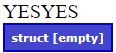`<``cfscript``>`
`CF9 = ``'Centaur'`
`CF10 = ``'Zeus'`
`CF11 = ``'Splendor'`
`CF2016 = ``'Raijin'`
`CF2018 = ``'Aether'`
`CF2021 = ``'Project Stratus'`

`CFReleaseCodeNames = \${`
`CF9,`
`CF10,`
`CF11,`
`CF2016,`
`CF2018,`
`CF2021`
`}`
`writeDump(CFReleaseCodeNames);`
`</``cfscript``>`

The Output of the above program will be as shown below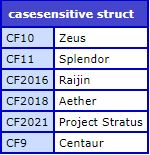Note: In the above examples, while declaring the Objects we have just defined keys and the values will be automatically taken up from the values contained in the keys.
2020-12-21 02:46:14

So pretty interesting and I’ll admit that some of this went over my head and made me think hard about what was going on.  I’d be interested in seeing a real world example of how you would use this if you were able to put one together.  This is pretty abstract and I think it’d help me grasp it more firmly seeing it in a real world scenario.  That being said I think I can see how I may could use this so will start trying to play.

Also I am assuming this is CF2021 specific?  On CFFiddle the code would only run with 2021 selected so may be worthy of noting in the description unless I am incorrect that is.

Thanks

>
Grae Desmond
's comment
2020-12-21 03:24:04
2020-12-21 03:24:04
>
Grae Desmond
's comment

Grae, yep, this is new to cf2021. And I can relate to your sentiment, having felt similarly when I first saw the feature and similar examples in the pre-release.

Fwiw, I will say that the feature is a generic one, and if you Google on it you’ll find it’s supported by other languages like Javascript, Python, etc.

And if you find resources on using the feature with those, the examples are similar, showing “how it works” but not so much “why would one bother”. I suspect they figure people can be left to connect the dots for the various ways one might leverage the capabilities.

But fwiw, as I kept looking for anything more, I did find this which you may appreciate :

https://www.smashingmagazine.com/2019/09/reintroduction-destructuring-assignment/

No, it’s not cf-specific, but again neither is the feature. Hope it may help.